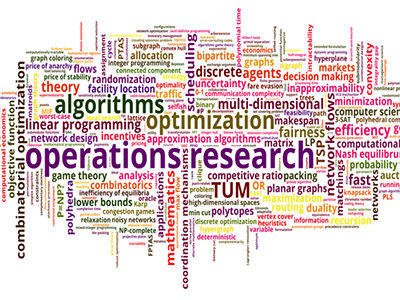ΓραμματείαSecretariat: 2410 684574 | ΦοιτητικάStudents: 2410 684387 g-ds@uth.gr
Select Page

# Operational Research

Ε803

8

#### Hours/Week - ECTS

4 – 5### Learning Outcomes

Upon successful completion of the course, the student will be able to:

• Know/state mathematical theory (concepts, theorems, proofs) which related to the course in question.
• Recognize the mathematical formulas related to the theory and to describe the solution methods (e.g. Method M)
• Distinguish different cases of linear problems programming and explain their use in relation to the given mathematical problem. (e.g. Binary theory, the transference problem.)
• Compute basic elements of the theory (e.g. solution via the Simplex technique).
• Understand, distinguish, and resolve the different cases of the issues that concern integer programming (Branch and bound, the partition problem, the minimum set overlap problem, dynamic programming, the knapsack problem (generalized knapsack)
• Fix problems with the local search method (e.g. Neighborhood Structure, methods neighborhood search, the street vendor problem, graph partitioning.)
• Explain and solve problems using Heuristic algorithms (Techniques performance evaluation, reachability ratio, the nodal overlap problem (vertex covering), maximum independent subset, upper and lower bounds, empirical evaluation of heuristics).
• Combine mathematical theory with problems of an economic nature that fall within in the course and to successfully proceed to exemplify them.

### Indicative Module Content

Business research models, complexity of algorithms, NP-hard problems. Linear programming: Simplex algorithm, Binary theory, the problem transportation. Integer programming: Branch and bound, the partition problem, the minimum set overlap problem, dynamic programming, the knapsack problem, generalized knapsack. Heuristic algorithms: Performance evaluation techniques, reachability ratio, the vertex covering problem, maximum independent subset, upper and lower bounds, empirical evaluation of heuristic methods. Local search methods: Neighborhood structure, neighborhood search methods, the traveling salesperson problem, graph partitioning. Simulated annealing: The Metropolis algorithm, applications, the maximum intersection problem.